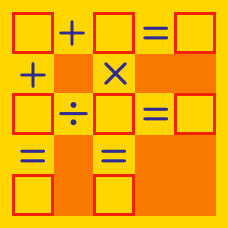Logic

# Hanging Balances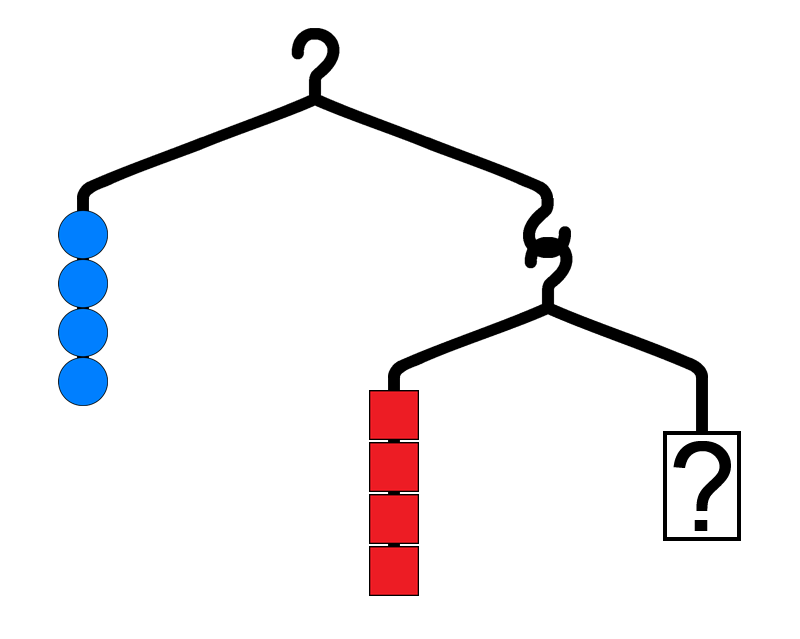In the above diagram, how many blue circles should go in the ? to make it balance?

Note: Assume the hangers and wire are all weightless and each hanger is symmetric.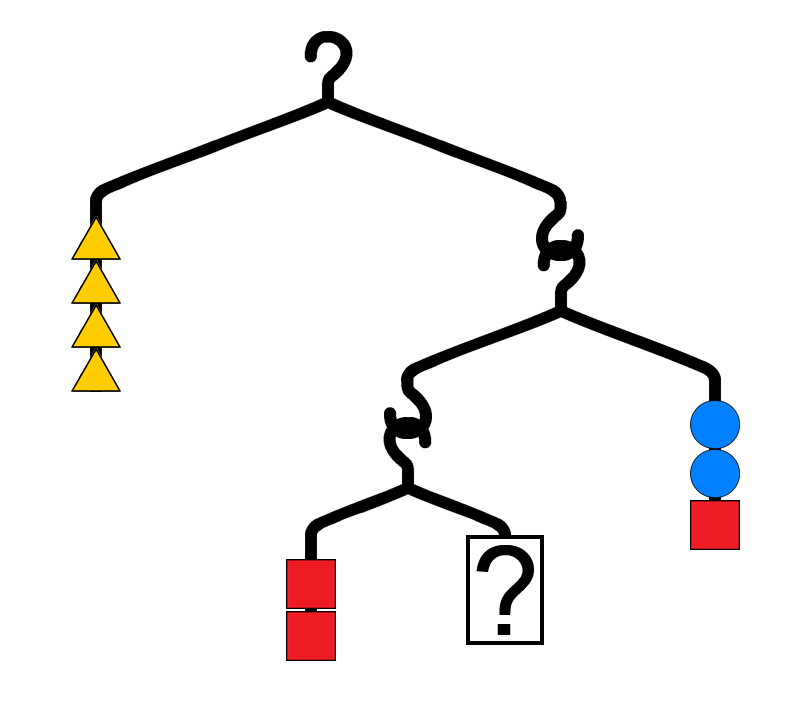In the above diagram, how many yellow triangles should go in the ? to make it balance?

Note: Assume the hangers and wire are all weightless and each hanger is symmetric.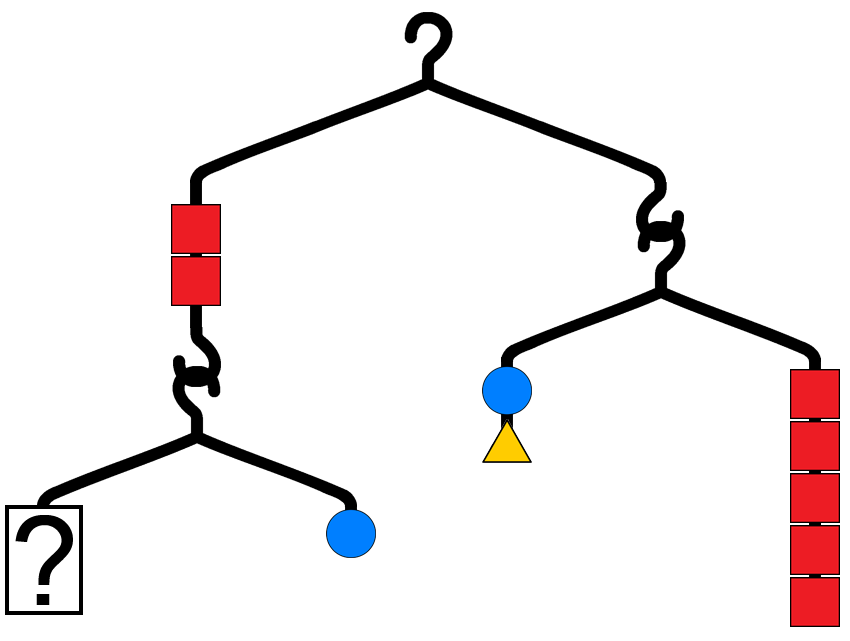In the above diagram, how many red squares should go in the ? to make it balance?

Note: Assume the hangers and wire are all weightless and each hanger is symmetric.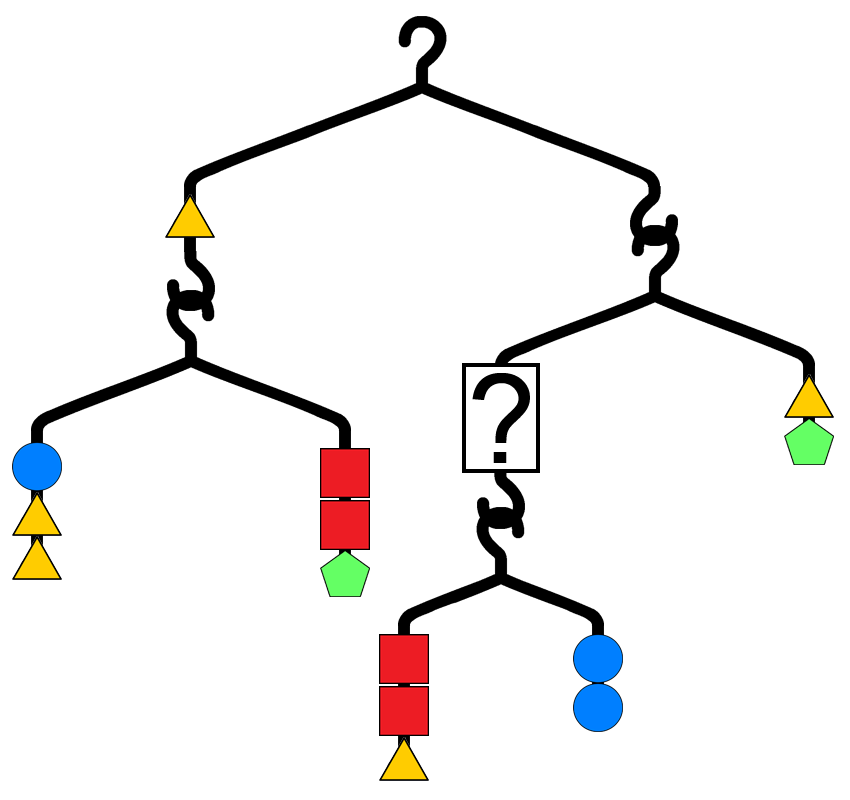In the above diagram, what should go in the ? to make it balance?

Note: Assume the hangers and wire are all weightless and each hanger is symmetric.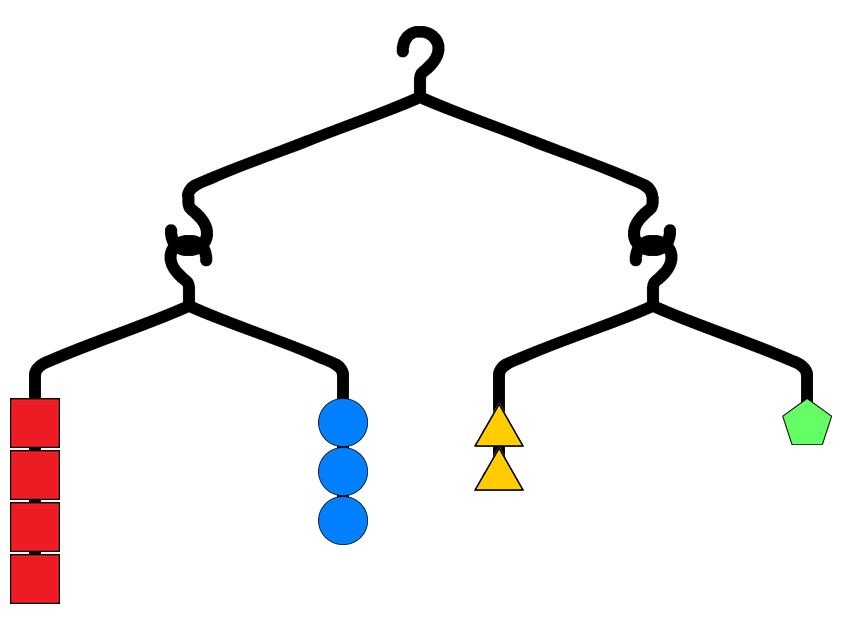According to the diagram above, one green pentagon weighs as much as how many red squares?

Note: Assume the hangers and wire are all weightless and each hanger is symmetric.

×

Problem Loading...

Note Loading...

Set Loading...